# Partition Equal Subset Sum (DP- 15)

Problem Link: Partition Equal Subset Sum

We are given an array ‘ARR’ with N positive integers. We need to find if we can partition the array into two subsets such that the sum of elements of each subset is equal to the other.

If we can partition, return true else return false

```Example: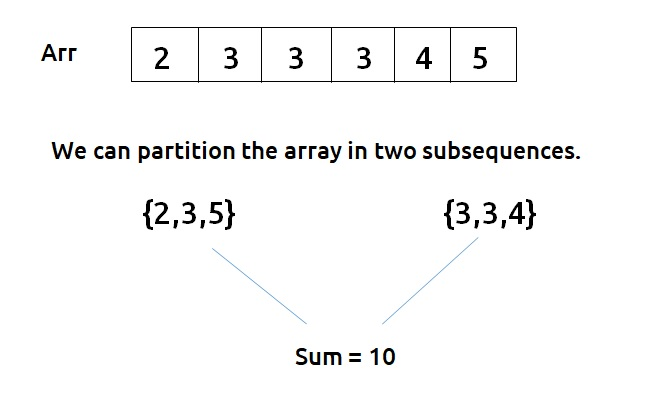```

In this example, as we are able to partition, we return true.

Disclaimer: Don’t jump directly to the solution, try it out yourself first.

### Solution :

This question is a slight modification of the problem discussed in Subset-sum equal to target. We need to partition the array(say S) into two subsets(say S1 and S2). According to the question:

• Sum of elements of S1 + sum of elements of S2 = sum of elements of S.
• Sum of elements of S1 = sum of elements of S2.

These two conditions imply that S1 = S2 = (S/2).

Now,

• If S (sum of elements of the input array) is odd , there is no way we can divide it into two equal halves, so we can simply return false.
• If S is even, then we need to find a subsequence in the input array whose sum is equal to S/2 because if we find one subsequence with sum S/2, the remaining elements sum will be automatically S/2. So, we can partition the given array. Hence we return true.

Note: Readers are highly advised to watch this video “Recursion on Subsequences” to understand how we generate subsequences using recursion.

From here we will try to find a subsequence in the array with target = S/2 as discussed in Subset-sum equal to the target

Steps to form the recursive solution:

We will first form the recursive solution by the three points mentioned in the Dynamic Programming Introduction

Step 1: Express the problem in terms of indexes.

The array will have an index but there is one more parameter “target”. We are given the initial problem to find whether there exists in the whole array a subsequence whose sum is equal to the target.

So, we can say that initially, we need to find(n-1, target) which means that we need to find whether there exists a subsequence in the array from index 0 to n-1, whose sum is equal to the target. Similarly, we can generalize it for any index ind as follows: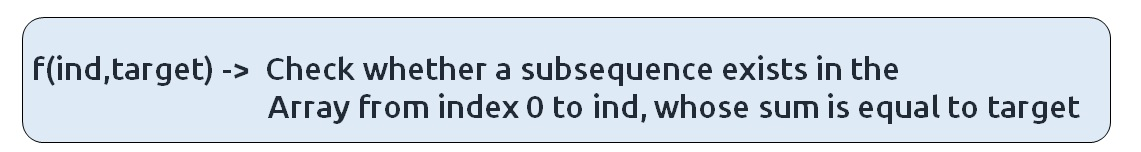Base Cases:

• If target == 0, it means that we have already found the subsequence from the previous steps, so we can return true.
• If ind==0, it means we are at the first element, so we need to return arr[ind]==target. If the element is equal to the target we return true else false.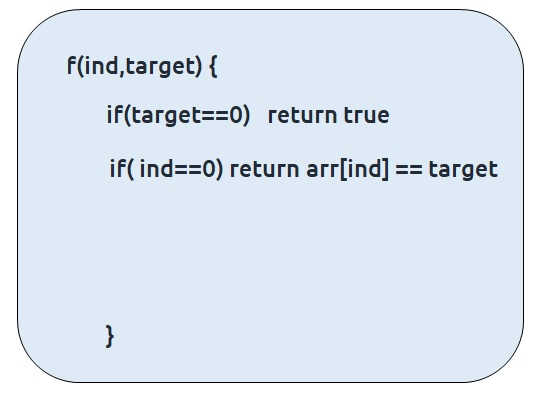Step 2: Try out all possible choices at a given index.

We need to generate all the subsequences. We will use the pick/non-pick technique as discussed in this video “Recursion on Subsequences”.

We have two choices:

• Exclude the current element in the subsequence: We first try to find a subsequence without considering the current index element. For this, we will make a recursive call to f(ind-1,target).
• Include the current element in the subsequence: We will try to find a subsequence by considering the current index as element as part of subsequence. As we have included arr[ind], the updated target which we need to find in the rest if the array will be target – arr[ind]. Therefore, we will call f(ind-1,target-arr[ind]).

Note: We will consider the current element in the subsequence only when the current element is less or equal to the target.

Step 3:  Return (taken || notTaken)

As we are looking for only one subset, if any of the one among taken or not taken returns true, we can return true from our function. Therefore, we return ‘or(||)’ of both of them.

The final pseudocode after steps 1, 2, and 3:

Steps to memoize a recursive solution:

If we draw the recursion tree, we will see that there are overlapping subproblems. In order to convert a recursive solution the following steps will be taken:

1. Create a dp array of size [n][k+1]. The size of the input array is ‘n’, so the index will always lie between ‘0’ and ‘n-1’. The target can take any value between ‘0’ and ‘k’. Therefore we take the dp array as dp[n][k+1]
2. We initialize the dp array to -1.
3. Whenever we want to find the answer of particular parameters (say f(ind,target)), we first check whether the answer is already calculated using the dp array(i.e dp[ind][target]!= -1 ). If yes, simply return the value from the dp array.
4. If not, then we are finding the answer for the given value for the first time, we will use the recursive relation as usual but before returning from the function, we will set dp[ind][target] to the solution we get.

Code:

## C++ Code

``````#include <bits/stdc++.h>

using namespace std;

bool subsetSumUtil(int ind, int target, vector<int>& arr, vector<vector<int>> &dp){
if(target==0)
return true;

if(ind == 0)
return arr == target;

if(dp[ind][target]!=-1)
return dp[ind][target];

bool notTaken = subsetSumUtil(ind-1,target,arr,dp);

bool taken = false;
if(arr[ind]<=target)
taken = subsetSumUtil(ind-1,target-arr[ind],arr,dp);

return dp[ind][target]= notTaken||taken;
}

bool canPartition(int n, vector<int> &arr){

int totSum=0;

for(int i=0; i<n;i++){
totSum+= arr[i];
}

if (totSum%2==1) return false;

else{
int k = totSum/2;
vector<vector<int>> dp(n,vector<int>(k+1,-1));
return subsetSumUtil(n-1,k,arr,dp);
}
}

int main() {

vector<int> arr = {2,3,3,3,4,5};
int n = arr.size();

if(canPartition(n,arr))
cout<<"The Array can be partitioned into two equal subsets";
else
cout<<"The Array cannot be partitioned into two equal subsets";
}
``````

Output:

The array can be partitioned into two equal subsets

Time Complexity: O(N*K) + O(N)

Reason: There are N*K states therefore at max ‘N*K’ new problems will be solved and we are running a for loop for ‘N’ times to calculate the total sum

Space Complexity: O(N*K) + O(N)

Reason: We are using a recursion stack space(O(N)) and a 2D array ( O(N*K)).

## Java Code

``````import java.util.*;

class TUF{
static boolean subsetSumUtil(int ind, int target,int arr[],int[][] dp){
if(target==0)
return true;

if(ind == 0)
return arr == target;

if(dp[ind][target]!=-1)
return dp[ind][target]==0?false:true;

boolean notTaken = subsetSumUtil(ind-1,target,arr,dp);

boolean taken = false;
if(arr[ind]<=target)
taken = subsetSumUtil(ind-1,target-arr[ind],arr,dp);
dp[ind][target]=notTaken||taken?1:0;
return notTaken||taken;
}

static boolean canPartition(int n,int[] arr){

int totSum=0;

for(int i=0; i<n;i++){
totSum+= arr[i];
}

if (totSum%2==1) return false;

else{
int k = totSum/2;
int dp[][]=new int[n][k+1];
for(int row[]: dp)
Arrays.fill(row,-1);
return subsetSumUtil(n-1,k,arr,dp);
}
}

public static void main(String args[]) {

int arr[] = {2,3,3,3,4,5};
int n = arr.length;

if(canPartition(n,arr))
System.out.println("The Array can be partitioned into two equal subsets");
else
System.out.println("The Array cannot be partitioned into two equal subsets");
}
}``````

Output:

The array can be partitioned into two equal subsets

Time Complexity: O(N*K) + O(N)

Reason: There are N*K states therefore at max ‘N*K’ new problems will be solved and we are running a for loop for ‘N’ times to calculate the total sum

Space Complexity: O(N*K) + O(N)

Reason: We are using a recursion stack space(O(N)) and a 2D array ( O(N*K)).

Steps to convert Recursive Solution to Tabulation one.

To convert the memoization approach to a tabulation one, create a dp array with the same size as done in memoization. We can set its type as bool and initialize it as false.First, we need to initialize the base conditions of the recursive solution.

• If target == 0, ind can take any value from 0 to n-1, therefore we need to set the value of the first column as true.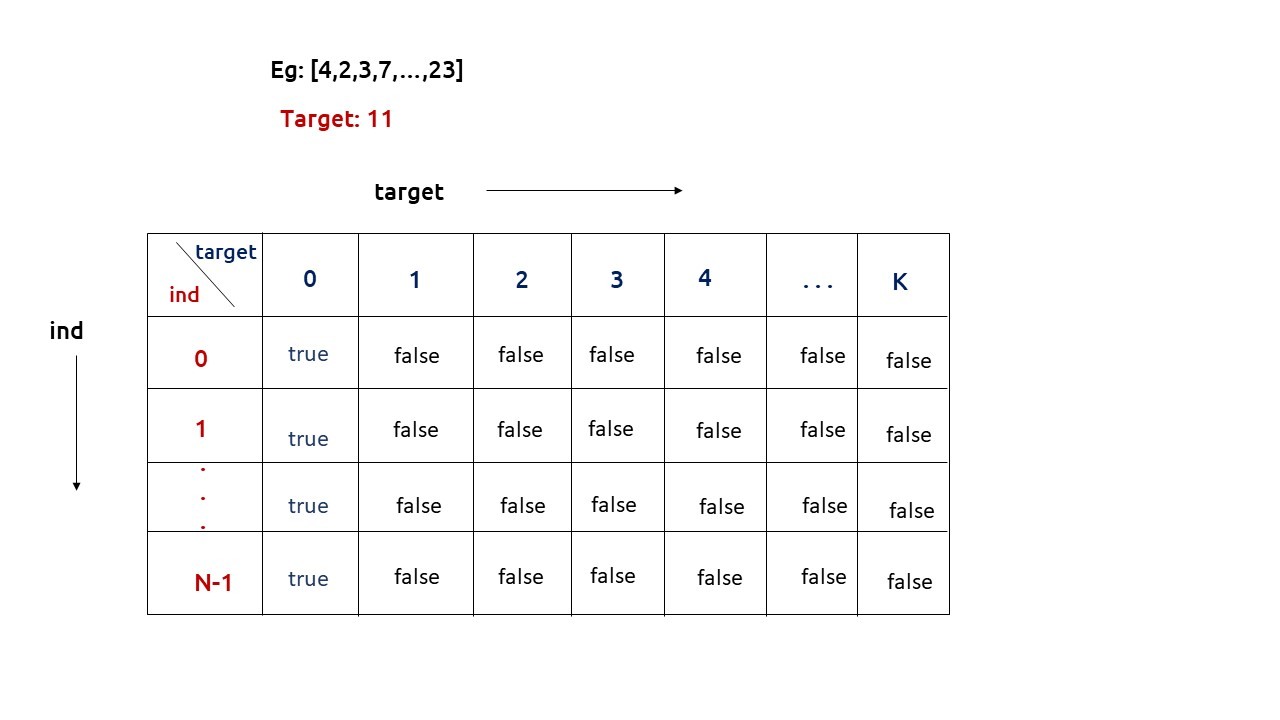• The first row dp[] indicates that only the first element of the array is considered, therefore for the target value equal to arr, only cell with that target will be true, so explicitly set dp[arr] =true, (dp[arr] means that we are considering the first element of the array with the target equal to the first element itself). Please note that it can happen that arr>target, so we first check it: if(arr<=target) then set dp[arr] = true.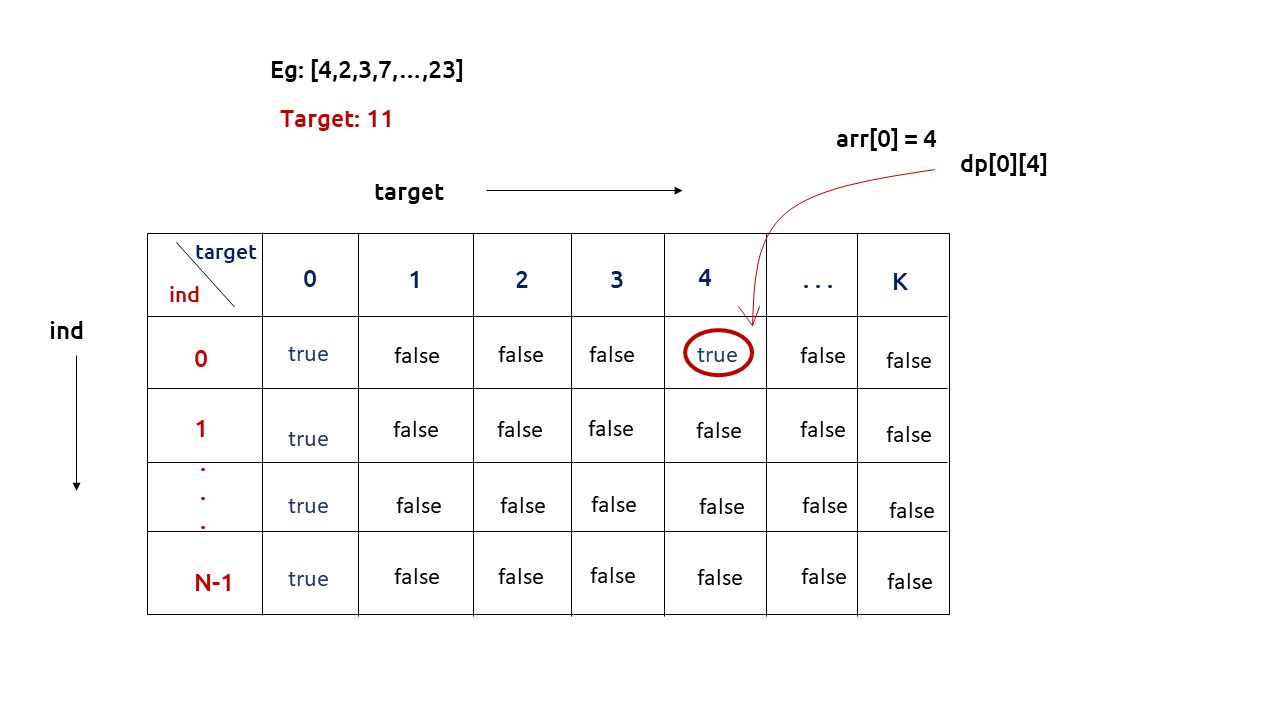• After that , we will set our nested for loops to traverse the dp array and following the logic discussed in the recursive approach, we will set the value of each cell. Instead of recursive calls, we will use the dp array itself.
• At last we will return dp[n-1][k] as our answer.

Code:

## C++ Code

``````#include <bits/stdc++.h>
using namespace std;

bool canPartition(int n, vector<int> &arr){

int totSum=0;

for(int i=0; i<n;i++){
totSum+= arr[i];
}

if (totSum%2==1) return false;

else{
int k = totSum/2;
vector<vector<bool>> dp(n,vector<bool>(k+1,false));

for(int i=0; i<n; i++){
dp[i] = true;
}

if(arr<=k)
dp[arr] = true;

for(int ind = 1; ind<n; ind++){
for(int target= 1; target<=k; target++){

bool notTaken = dp[ind-1][target];

bool taken = false;
if(arr[ind]<=target)
taken = dp[ind-1][target-arr[ind]];

dp[ind][target]= notTaken||taken;
}
}

return dp[n-1][k];

}
}

int main() {

vector<int> arr = {2,3,3,3,4,5};
int n = arr.size();

if(canPartition(n,arr))
cout<<"The Array can be partitioned into two equal subsets";
else
cout<<"The Array cannot be partitioned into two equal subsets";
}
``````

Output:

The array can be partitioned into two equal subsets

Time Complexity: O(N*K) +O(N)

Reason: There are two nested loops that account for O(N*K) and at starting we are running a for loop to calculate totSum.

Space Complexity: O(N*K)

Reason: We are using an external array of size ‘N*K’. Stack Space is eliminated.

## Java Code

``````import java.util.*;

class TUF{

static boolean canPartition(int n,int[] arr){

int totSum=0;

for(int i=0; i<n;i++){
totSum+= arr[i];
}

if (totSum%2==1) return false;

else{
int k = totSum/2;
boolean dp[][]=new boolean[n][k+1];

for(int i=0; i<n; i++){
dp[i] = true;
}

if(arr<=k)
dp[arr] = true;

for(int ind = 1; ind<n; ind++){
for(int target= 1; target<=k; target++){

boolean notTaken = dp[ind-1][target];

boolean taken = false;
if(arr[ind]<=target)
taken = dp[ind-1][target-arr[ind]];

dp[ind][target]= notTaken||taken;
}
}

return dp[n-1][k];

}
}

public static void main(String args[]) {

int arr[] = {2,3,3,3,4,5};
int n = arr.length;

if(canPartition(n,arr))
System.out.println("The Array can be partitioned into two equal subsets");
else
System.out.println("The Array cannot be partitioned into two equal subsets");
}
}``````

Output:

The array can be partitioned into two equal subsets

Time Complexity: O(N*K) +O(N)

Reason: There are two nested loops that account for O(N*K) and at starting we are running a for loop to calculate totSum.

Space Complexity: O(N*K)

Reason: We are using an external array of size ‘N*K’. Stack Space is eliminated.

Part 3: Space Optimization

If we closely look the relation,

dp[ind][target] =  dp[ind-1][target] || dp[ind-1][target-arr[ind]]

We see that to calculate a value of a cell of the dp array, we need only the previous row values (say prev). So, we don’t need to store an entire array. Hence we can space optimize it.

Note: Whenever we create a new row ( say cur), we need to explicitly set its first element is true according to our base condition.

Code:

## C++ Code

``````#include <bits/stdc++.h>

using namespace std;

bool canPartition(int n, vector<int> &arr){

int totSum=0;

for(int i=0; i<n;i++){
totSum+= arr[i];
}

if (totSum%2==1) return false;

else{
int k = totSum/2;
vector<bool> prev(k+1,false);

prev = true;

if(arr<=k)
prev[arr] = true;

for(int ind = 1; ind<n; ind++){
vector<bool> cur(k+1,false);
cur = true;
for(int target= 1; target<=k; target++){
bool notTaken = prev[target];

bool taken = false;
if(arr[ind]<=target)
taken = prev[target-arr[ind]];

cur[target]= notTaken||taken;
}
prev = cur;
}

return prev[k];
}
}

int main() {

vector<int> arr = {2,3,3,3,4,5};
int n = arr.size();

if(canPartition(n,arr))
cout<<"The Array can be partitioned into two equal subsets";
else
cout<<"The Array cannot be partitioned into two equal subsets";
}
``````

Output:

The Array can be partitioned into two equal subsets

Time Complexity: O(N*K) +O(N)

Reason: There are two nested loops that account for O(N*K) and at starting we are running a for loop to calculate totSum.

Space Complexity: O(K)

Reason: We are using an external array of size ‘K+1’ to store only one row.

## Java Code

``````import java.util.*;

class TUF{

static boolean canPartition(int n,int[] arr){

int totSum=0;

for(int i=0; i<n;i++){
totSum+= arr[i];
}

if (totSum%2==1) return false;

else{
int k = totSum/2;
boolean prev[]=new boolean[k+1];

prev = true;

if(arr<=k)
prev[arr] = true;

for(int ind = 1; ind<n; ind++){
boolean cur[]=new boolean[k+1];
cur = true;
for(int target= 1; target<=k; target++){
boolean notTaken = prev[target];

boolean taken = false;
if(arr[ind]<=target)
taken = prev[target-arr[ind]];

cur[target]= notTaken||taken;
}
prev = cur;
}

return prev[k];
}
}

public static void main(String args[]) {

int arr[] = {2,3,3,3,4,5};
int n = arr.length;

if(canPartition(n,arr))
System.out.println("The Array can be partitioned into two equal subsets");
else
System.out.println("The Array cannot be partitioned into two equal subsets");
}
}``````

Output:

The Array can be partitioned into two equal subsets

Time Complexity: O(N*K) +O(N)

Reason: There are two nested loops that account for O(N*K) and at starting we are running a for loop to calculate totSum.

Space Complexity: O(K)

Reason: We are using an external array of size ‘K+1’ to store only one row.

Special thanks to Anshuman Sharma for contributing to this article on takeUforward. If you also wish to share your knowledge with the takeUforward fam, please check out this article